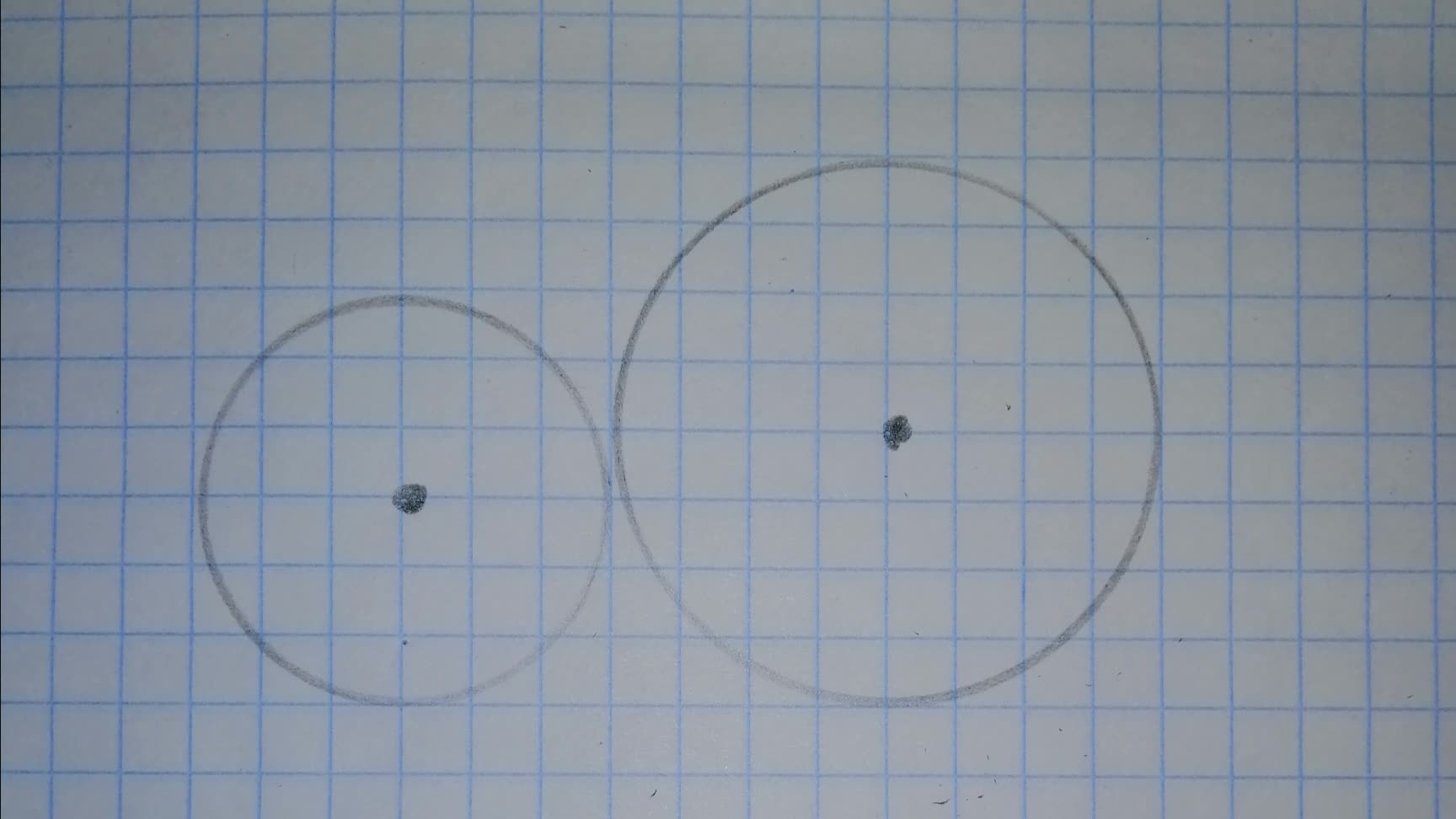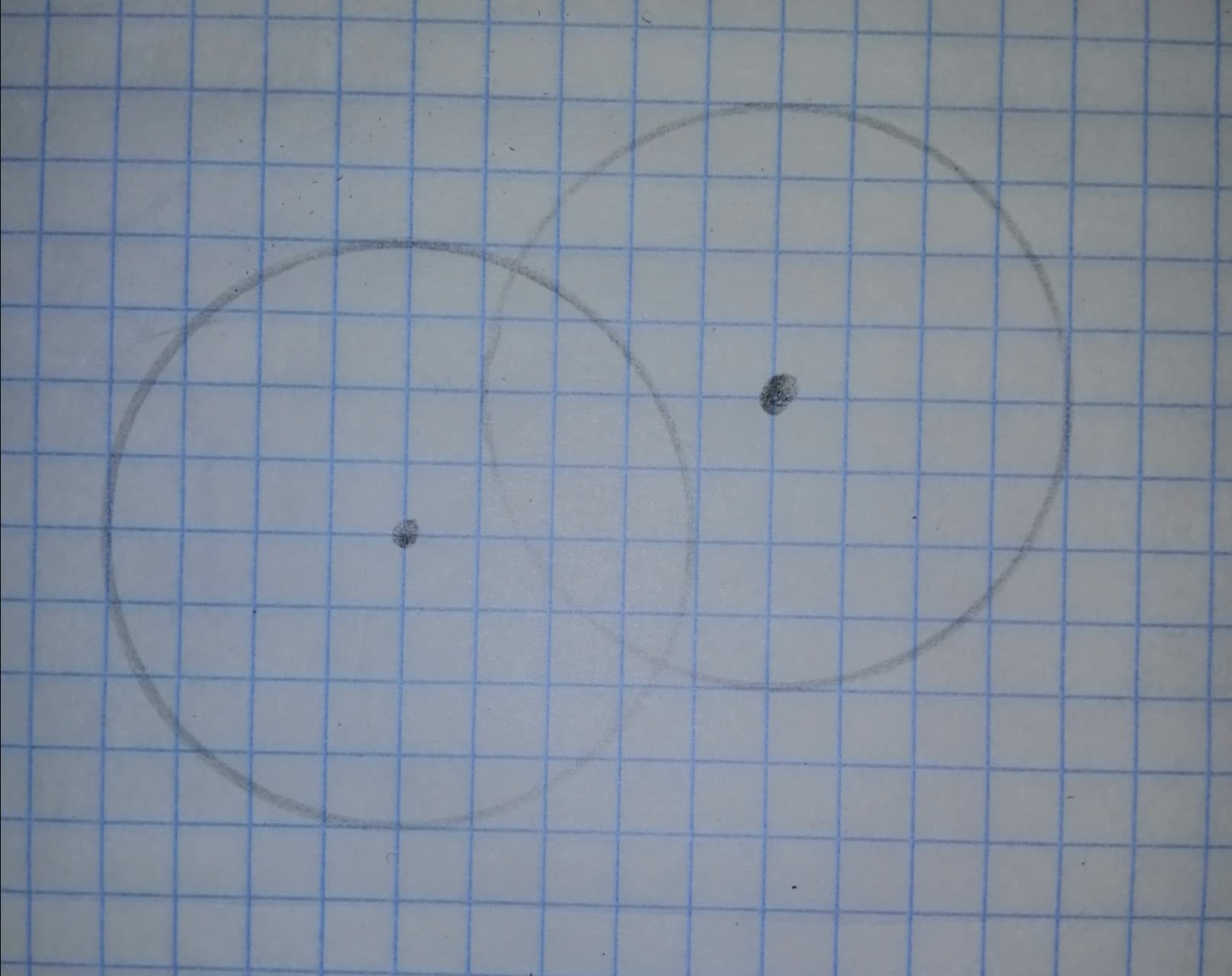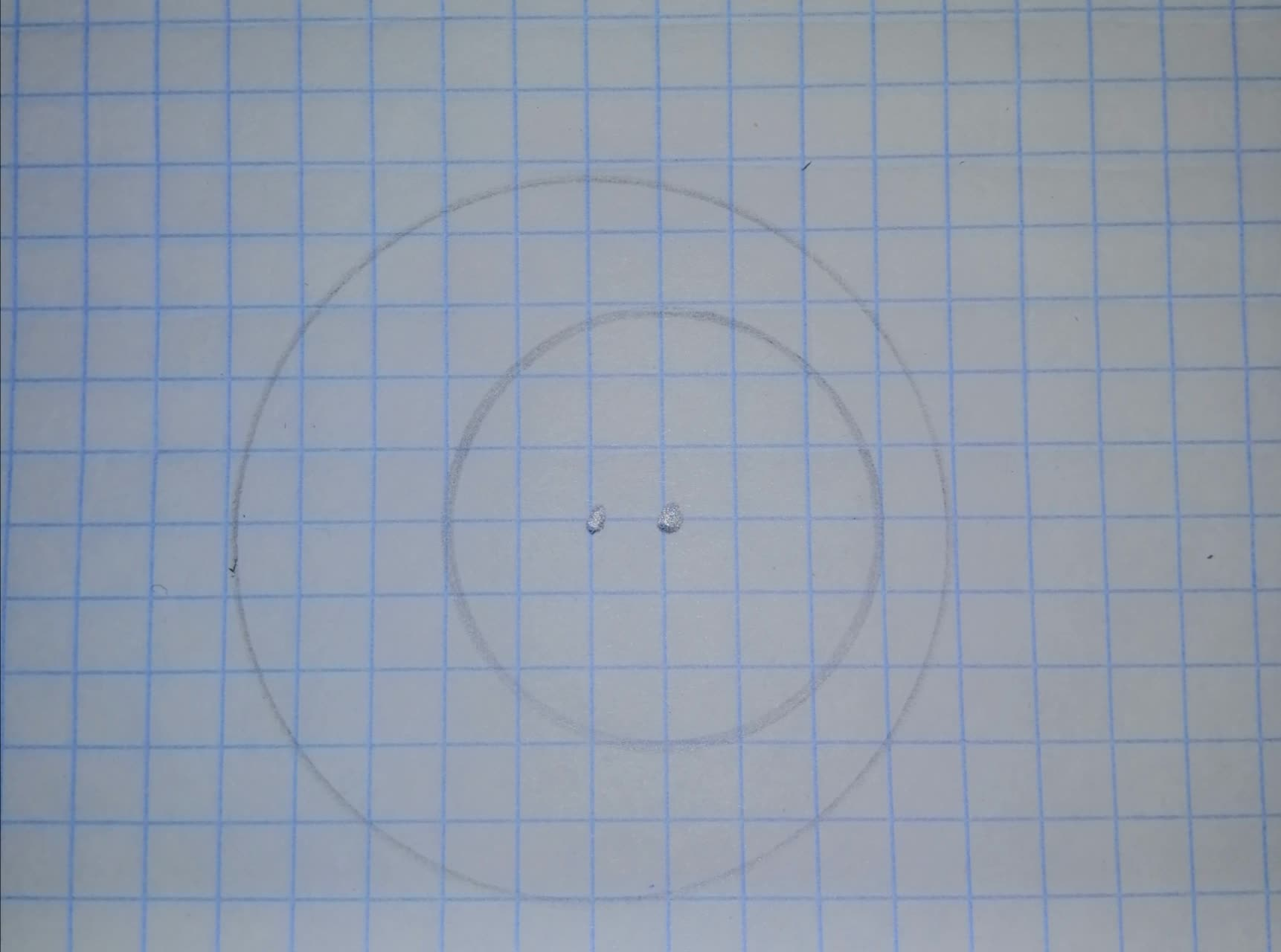Question# For the two circles in Figures (a), (b), and (c),

Circles
ANSWEREDFor the two circles in Figures (a), (b), and (c), find the total number of common tangents (internal and external).
a)b)c)2021-08-18

Step 1
We have to find
Total Number of common tangents (Internally and externally)
As we know,
Two circles in the same plane have Common Internal Tangent if they intersect in exactly one point and the intersection of their interiors is not empty.
Two circles in the same plane have Common External Tangent if they intersect in exactly one point and the intersection of their interiors is empty
Step 2
a)2 External Tangent
1 Internal Tangent
Step 3
b)c)